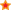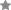for Secondary Education in the UK.Find Resources By Subjects
Information

# Catching up on Numeracy: Pencil and Paper Methods

Price: £25.00
Age Range: KS3

Address key skills and concepts in pencil and paper recording. Encourage students to develop a variety of strategies based on known mathematical facts. Subjects covered include squares, factors, multiples, decimals, fractions, and measurement of time, weight and distance.

Topics include: Exploring addition, subtraction, multiplication and division; Using short cuts; Rounding numbers; The contexts of money, weight, volume and time; Fractions, percentages and decimals. (Formerly: Added Value in Numeracy: Pencil and Paper methods)

Quantity Format
Size: 64 Pages
ISBN: 978 1 86025 226 6
Code: CNPP
Popularity rank: 126
Average Customer Review:based on 1 review

# Contents List

#### LESSONS

• 7 DIFFERENT WAYS A variety of effective ways to multiply numbers.
• 9 FIVES AND TENS Investigating the properties and uses of multiplication chains.
• 11 LINE UP Lining numbers up under one another to check addition.
• 13 HUNDREDS, TENS AND UNITS Addition and estimation.
• 15 TAKE AWAY Subtraction and estimation.
• 17 DIVIDE AND CHECK Simple division and checking answers by multiplication.
• 19 NUMBERS MAKING HALVES How to tell if a number can be divided by 2 or 4.
• 21 MOVE RIGHT How numbers behave when divided by 10, 100 and 1000.
• 23 POINT BLANK Using sets to identify numbers that will divide by 10, 100 and 1000.
• 25 TENS, HUNDREDS, THOUSANDS Multiplying numbers and decimals by 10, 100 and 1000.
• 27 TIMES TWENTY Students build on existing knowledge as they work with multiples of 10.
• 29 PERCENTAGES Finding percentages using pencil, paper and plenty of practical examples.
• 31 THE SALE Calculating percentages and deducting them from original prices.
• 33 CASH Adding and subtracting money.
• 35 CASH MULTIPLES AND SHARES Multiplying cash amounts.
• 37 METRES AND CENTIMETRES Converting metres into centimetres, and adding metric lengths.
• 39 HEAVY WEIGHT Adding and subtracting metric weights.
• 41 SIMPLE FRACTIONS Adding fractions using segment diagrams and by changing denominators.
• 43 FRACTION SUBTRACTION Subtracting fractions using segment diagrams and by changing denominators.
• 45 MONEY FRACTIONS Using fractions in financial calculations.
• 47 PRIME FACTORS Changing whole numbers into the products of primes.
• 49 FAST OR SLOW? Adding and subtracting units of time using pencil and paper.
• 51 DAY TRIP Calculating time intervals using pencil and paper.
• 55 DECIMAL TAKE-AWAY Subtracting numbers to two decimal places.
• 57 DECIMAL TIMES Multiplying decimal numbers by whole numbers.
• 59 DECIMAL DIVISION Dividing whole and decimal numbers by decimals up to two places.
• 61 GOES INTO Dividing financial amounts by whole numbers.
• 62 APPENDIX Bingo cards for page 7, DIFFERENT WAYS.

## Reviews

20/07/10

### Name and school withheld.

few new ideas, may use in lessons